# Digital Multimeter Working Principle

Want create site? Find Free WordPress Themes and plugins.

To test and troubleshoot circuits, it is necessary to understand how to use basic electronic measuring instruments. The digital multimeter (DMM) is the most widely used electronic measuring instrument. Also, the clamp-type ammeter is useful for measuring current, especially in power applications. An older analog meter called the volt-ohm-milliammeter (VOM) is still used in some applications but has largely been replaced by the DMM.

## Digital Multimeter Working

digital multimeter (DMM) is an instrument that can measure several basic electrical quantities and shows the measurement with a number in a display.

All multimeters measure ac and dc voltage (voltmeter), ac and dc current (ammeter), and resistance (ohmmeter). Some can also measure other electrical quantities such as frequency or even temperature. A typical DMM with probes is shown in Figure 1.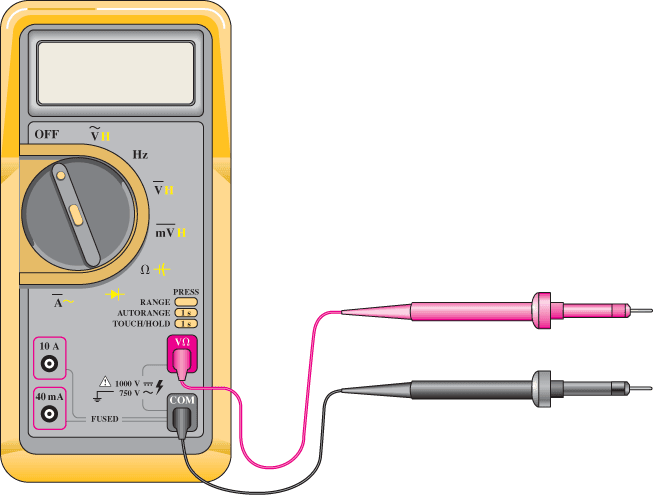Figure 1: Typical DMM with probes

## DC Voltage Measurements

To measure voltage, connect the meter leads across the component to be measured (this is a parallel connection). With digital meters, the meter normally sets the polarity automatically, and the sign is indicated on the display. It is convenient to use a red lead in the voltage jack and a black one on the common. Then positive or negative readings are easy to interpret.

Many DMMs are capable of auto-ranging, a feature in which the DMM selects the optimum range automatically for displaying the reading. The range is the maximum voltage that can be displayed with a particular setting. Lower-priced meters have manual ranging, which requires the user to select an appropriate range for the measurement.

To make a voltage measurement, first move the selector switch to DC VOLTS; if the meter is a manual-ranging type, choose a range larger than the expected voltage (this is not necessary with an auto-ranging meter). Sometimes the dc volts position is indicated by the letter V and a straight line over a dashed line (to remind you of dc). Only after selecting the proper function (DC VOLTS) and range, should you connect the meter to the circuit.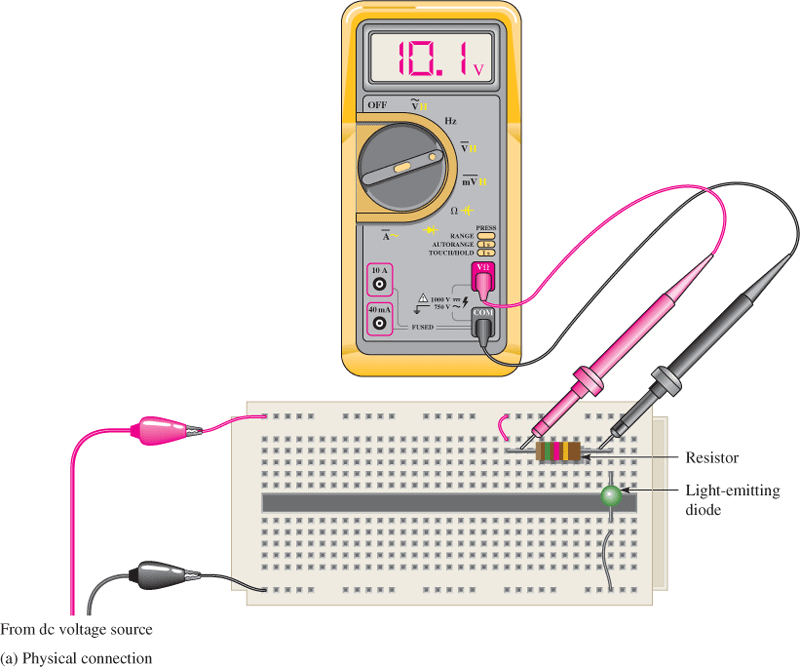Figure 2: DC Voltage Measurement.

The meter is connected directly across the component to be measured; in this case, the reading is the voltage across the resistor.

Most meters indicate the voltage no matter which way the leads are connected to the circuit. If the positive meter lead is connected to the more positive voltage, then the voltage reading is displayed as a positive value; otherwise, it is indicated as a negative value. An example of measuring the voltage in a basic circuit on a proto-board is shown in Figure 2(a). The schematic equivalent is shown in Figure 2(b).

## AC Voltage Measurements

AC voltage measurements are done in the same way as dc measurements except the selector switch must be in the AC VOLTS position. Some meters indicate ac with a small sine wave. As is the case with dc measurements, select the function and range (if necessary) before you hook the meter to the circuit. Because ac goes between positive and negative values, the polarity is not important and is displayed as a positive value in any case. It is important to realize that the reading is displayed as an RMS value for ac.

Another important consideration in ac voltage measurements is the frequency you are measuring. DMMs vary widely in their ability to measure higher frequencies; many are accurate only for a very small range of frequencies. Typically, the range may be from 45 Hz to 1 kHz (1 kilohertz), but some meters can be used up to 1 MHz (megahertz). You should check the accuracy specification of a meter.

## Current Measurements

For DMMs, select either the AC or DC function (it is necessary to move the probes to separate current jacks on the meter). As in any current measurement, connect the meter in series (in line) with the circuit under test. It is necessary to break a connection in the circuit in order to insert the meter. In this case, the meter is inserted in series between the voltage source and the resistor. Note that, if you are measuring ac, it will be shown on the display as the RMS value. A current measurement on a proto-board is shown in Figure 3(a); its schematic equivalent is illustrated in Figure 3(b).

Caution! If the meter is inadvertently connected across a voltage source, there will be very high current through the meter, causing either a fuse to blow or damage to the meter.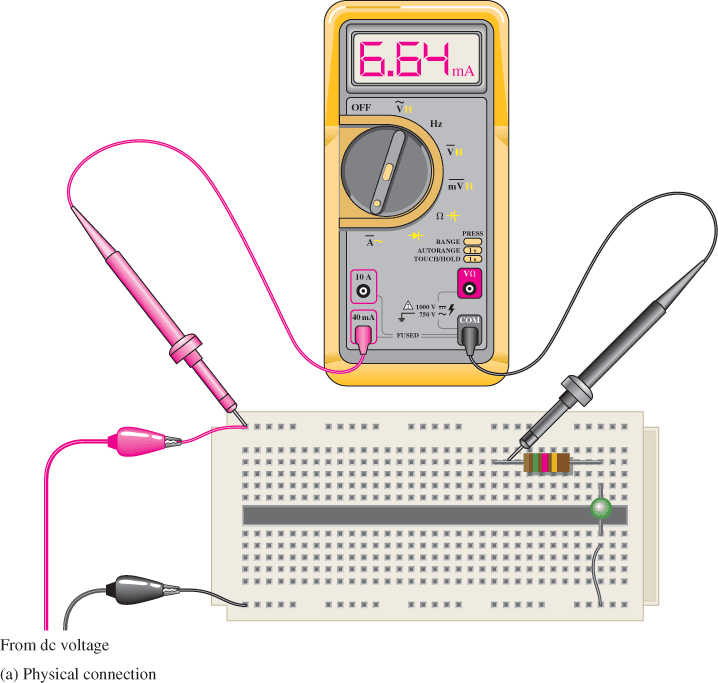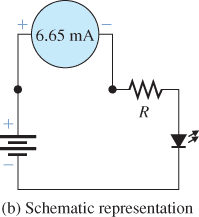Figure 3: DC Current Measurement. The meter is connected in series with the components by breaking the circuit. In this case, the reading is the current through the resistor and LED.

## Clamp Meter Working

A useful meter for measuring alternating and direct current is the clamp meter, which does not require the circuit to be broken to insert the meter. The meter may be a stand-alone unit or part of a traditional DMM. Figure 4(a) shows a typical clamp meter, and Figure 4(b) shows a technician using a clamp meter (shown held in the technician’s right hand). The sensing element is a set of jaws that can be opened and closed around a single conductor. The meter senses the magnetic field from the current and converts it directly to a current reading.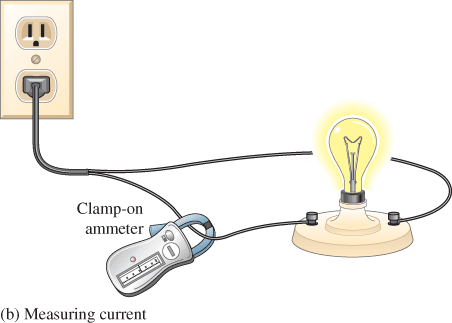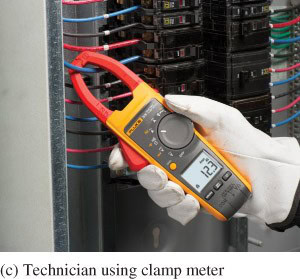Figure 4: Clamp Meter. The clamp meter makes it easy to measure current.

## Resistance Measurements

DMMs measure resistance by using an internal current source to provide an accurate, fixed current through the unknown resistance. This develops a voltage across the unknown resistance that is proportional to the resistance. The voltage developed across the unknown is converted internally by the meter to a resistance value and displayed.

Resistance is always measured with one (or both) ends of the resistor under test disconnected from the circuit to ensure that another path in the circuit or another voltage is not present that can cause the meter to produce an erroneous reading or damage the meter. Figure 5(a) illustrates a resistance reading on a proto-board. Its schematic equivalent is shown in Figure 5(b). Notice that one end of the resistor is disconnected from the source.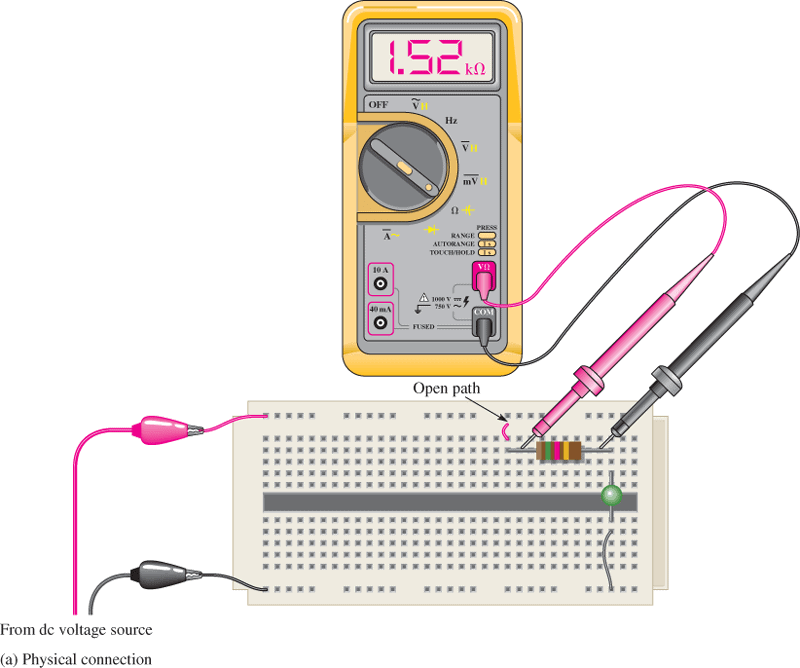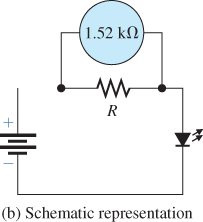Figure 5: Resistance Measurement. The meter is connected across the resistor after first disconnecting one end of the resistor. In this case, the reading is the resistance of the resistor.

Review Questions

1. What measurements can be made with a basic DMM?
2. What are two procedures that must be done when connecting a DMM for a current measurement?
3. Why is it necessary to disconnect a voltage source when using a DMM to measure resistance?
4. Is it easier to measure current with a DMM or a clamp-on ammeter?
5. What is a continuity check?